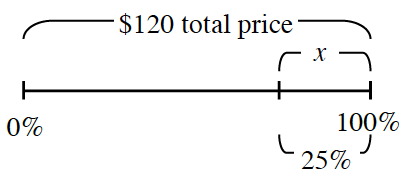### Home > CC2MN > Chapter 8 > Lesson 8.1.8 > Problem8-92

8-92.

The electronics department is having a No Sales Tax Sale! In addition, all of the items in the department are on sale for $25\%$ off. Wyatt is looking at a music player that normally costs $\120$. He has $\95$ to spend, and he is wondering if he has enough money to buy it.

1.Wyatt sketched the diagram at right. Use the work he started to find $25\%$ of $\120$. Is this the price he will pay?

Solve for $x$.

$\frac{25}{100} = \frac{\textit{x}}{120}$

2. Does Wyatt have enough money?

Solving for $x$ in part (a) only found the amount of the discount. What price will Wyatt actually have to pay?

3. Would he have enough money if he had to pay the $5.5\%$ sales tax on the sale price?

How much is $5.5\%$ of the price? Try using the Giant One method to solve for the amount of sales tax Wyatt will have to pay.

Yes, Wyatt would have enough money even if he had to pay a $5.5\%$ sales tax on the sale price.